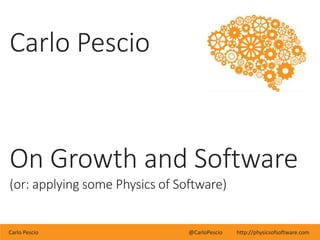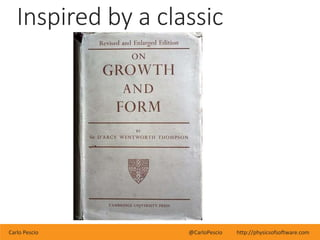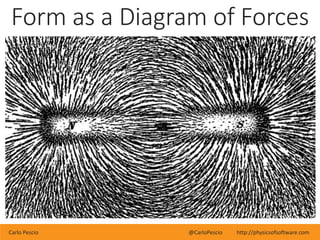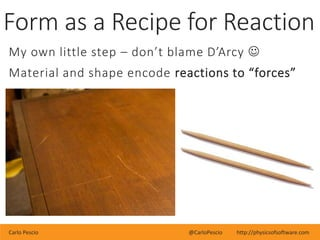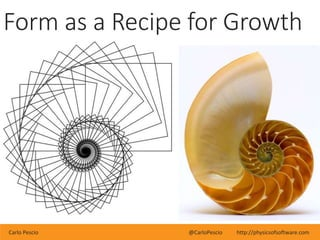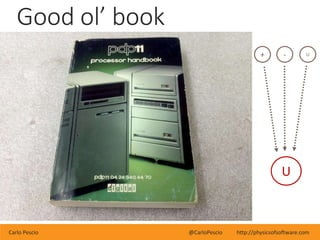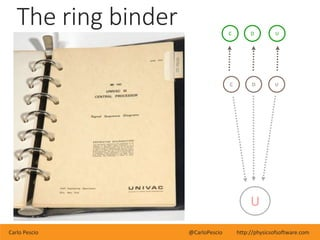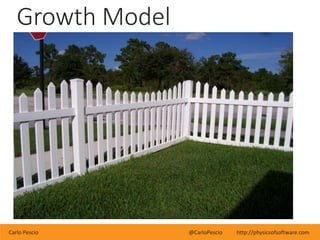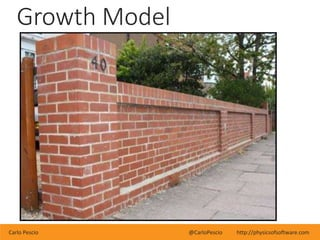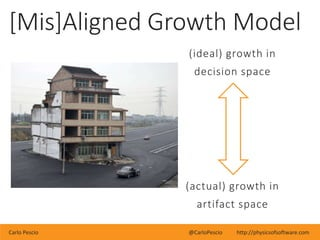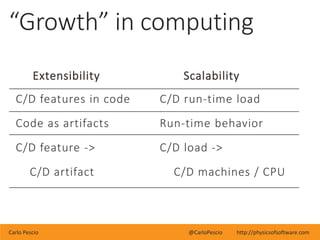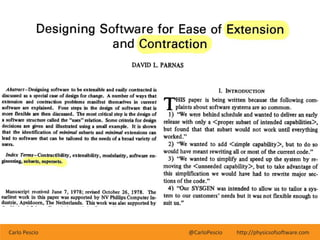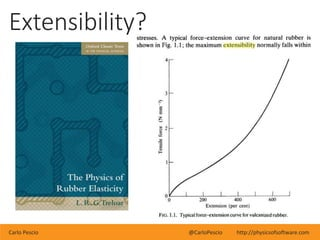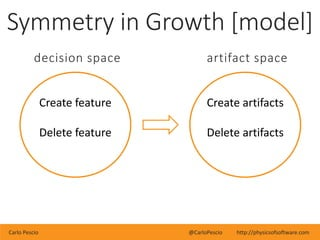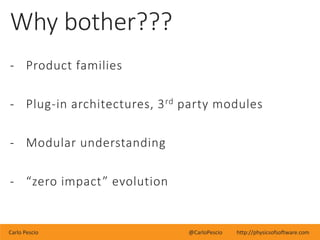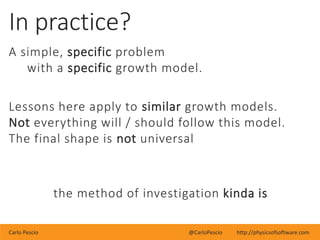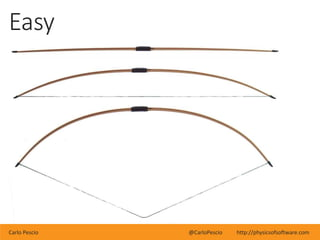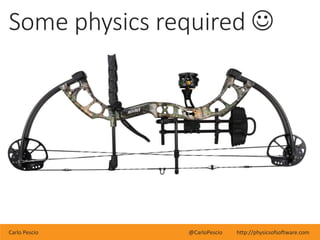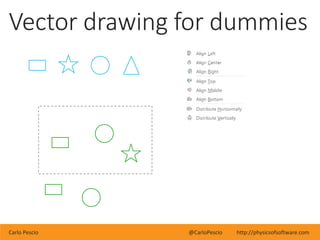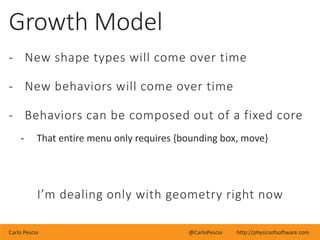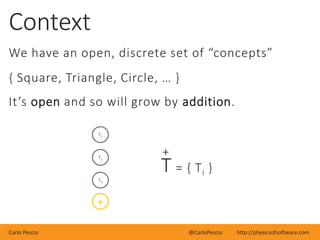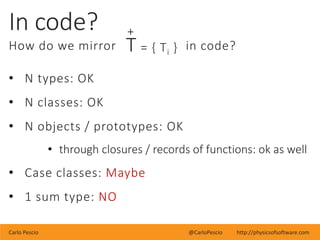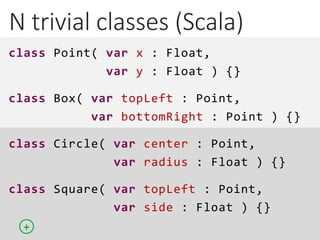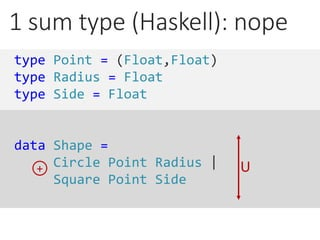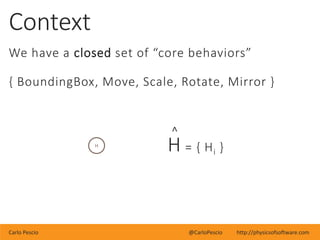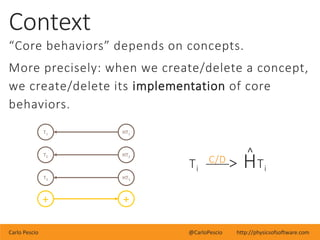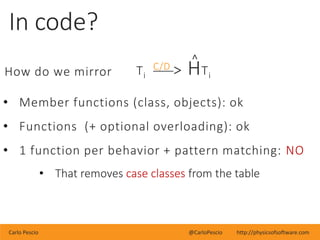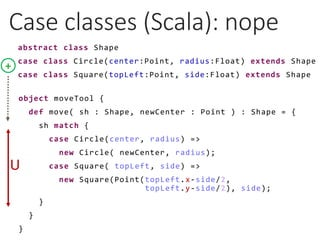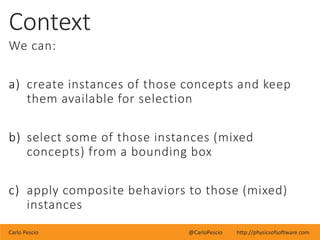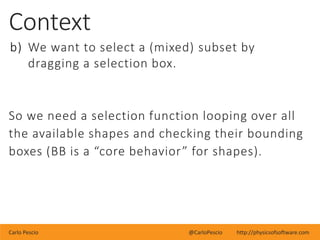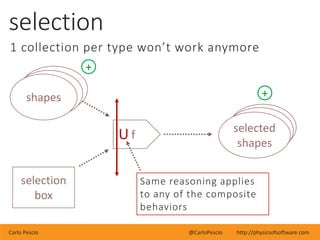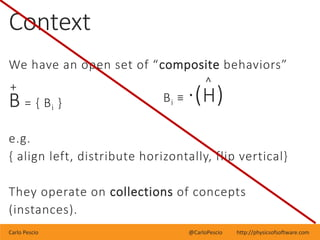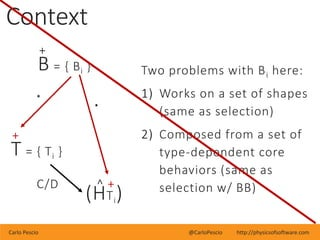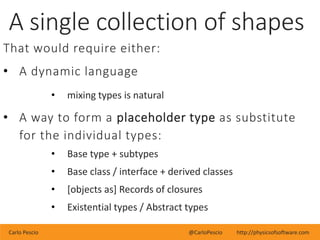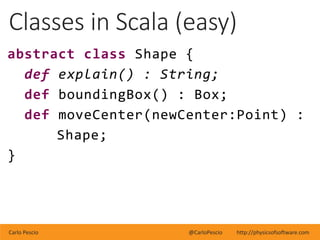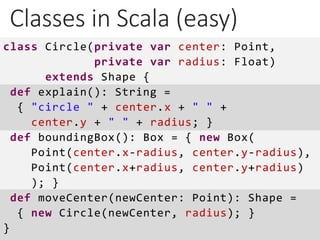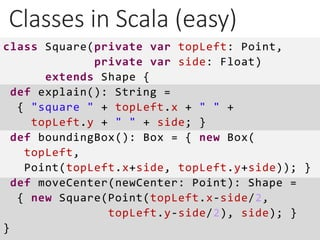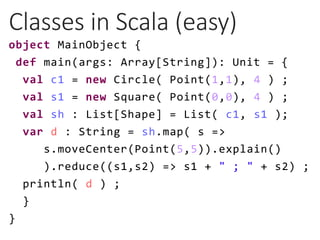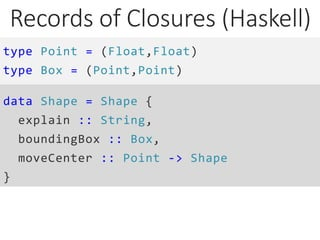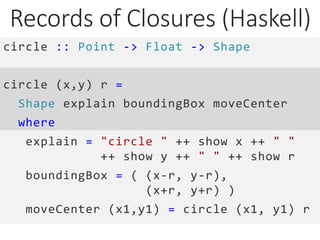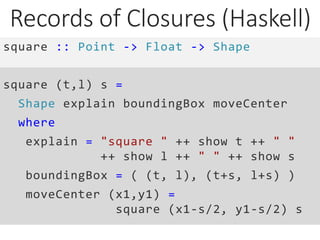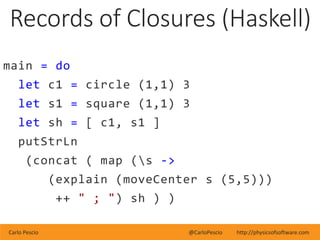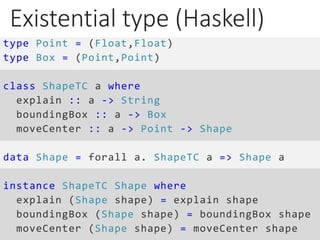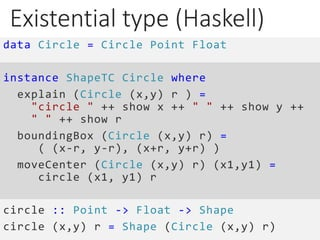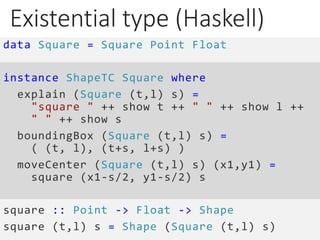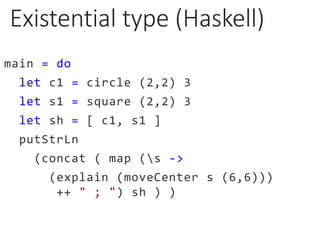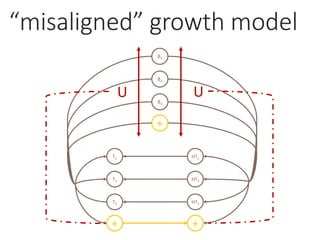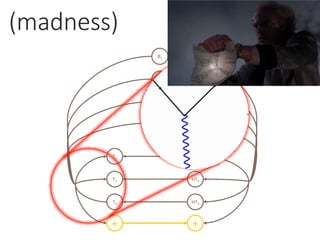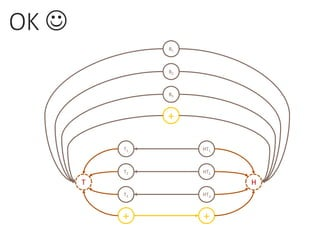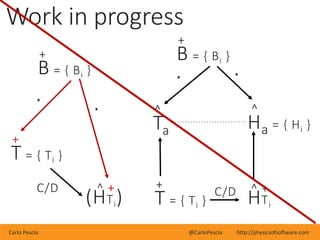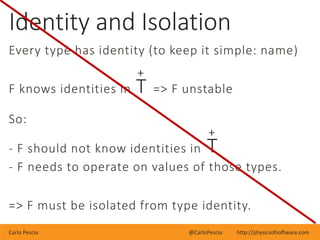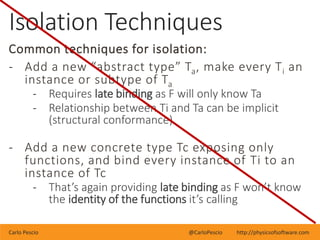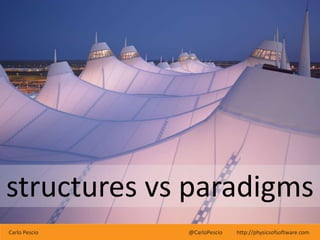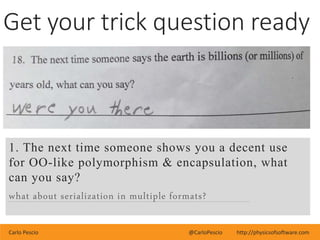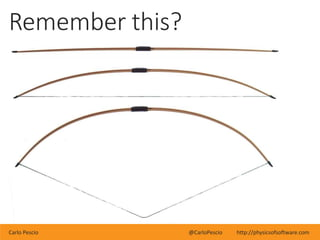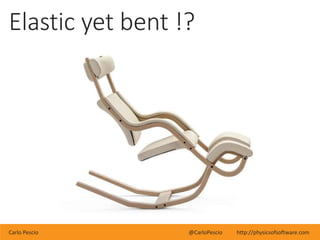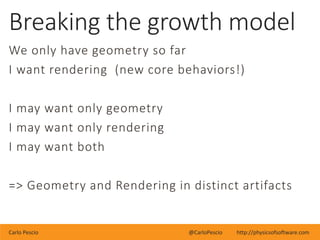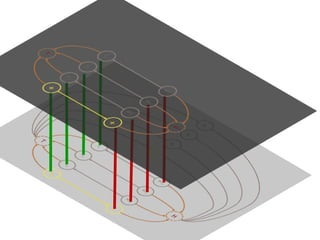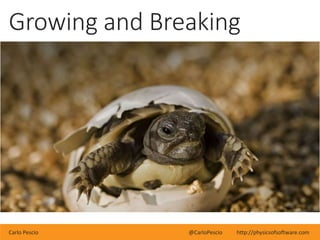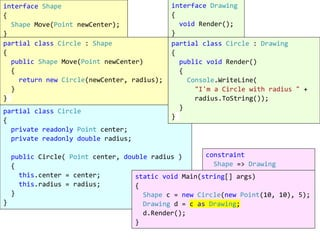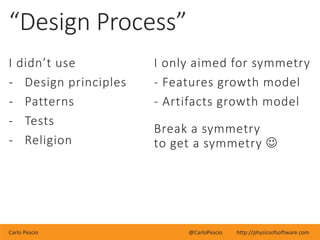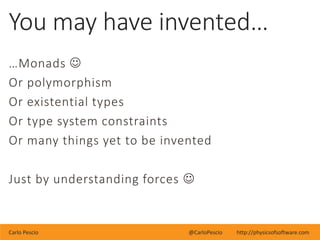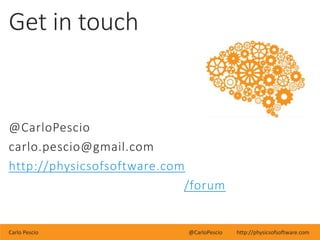1 of 65

### On Growth and Software

1. Carlo Pescio @CarloPescio http://physicsofsoftware.com Carlo Pescio On Growth and Software (or: applying some Physics of Software)
2. Carlo Pescio @CarloPescio http://physicsofsoftware.com Inspired by a classic
3. Carlo Pescio @CarloPescio http://physicsofsoftware.com Form as a Diagram of Forces
4. Carlo Pescio @CarloPescio http://physicsofsoftware.com Form as a Recipe for Reaction My own little step – don’t blame D’Arcy  Material and shape encode reactions to “forces”
5. Carlo Pescio @CarloPescio http://physicsofsoftware.com Form as a Recipe for Growth
6. Carlo Pescio @CarloPescio http://physicsofsoftware.com Good ol’ book + - U U
7. Carlo Pescio @CarloPescio http://physicsofsoftware.com The ring binder U D D U U C C
8. Carlo Pescio @CarloPescio http://physicsofsoftware.com Growth Model
9. Carlo Pescio @CarloPescio http://physicsofsoftware.com Growth Model
10. Carlo Pescio @CarloPescio http://physicsofsoftware.com [Mis]Aligned Growth Model (ideal) growth in decision space (actual) growth in artifact space
11. Carlo Pescio @CarloPescio http://physicsofsoftware.com “Growth” in computing Extensibility Scalability C/D features in code C/D run-time load Code as artifacts Run-time behavior C/D feature -> C/D load -> C/D artifact C/D machines / CPU
12. Carlo Pescio @CarloPescio http://physicsofsoftware.com
13. Carlo Pescio @CarloPescio http://physicsofsoftware.com Extensibility?
14. Carlo Pescio @CarloPescio http://physicsofsoftware.com Symmetry in Growth [model] Create feature Delete feature decision space Create artifacts Delete artifacts artifact space
15. Carlo Pescio @CarloPescio http://physicsofsoftware.com Why bother??? - Product families - Plug-in architectures, 3rd party modules - Modular understanding - “zero impact” evolution
16. Carlo Pescio @CarloPescio http://physicsofsoftware.com In practice? A simple, specific problem with a specific growth model. Lessons here apply to similar growth models. Not everything will / should follow this model. The final shape is not universal the method of investigation kinda is
17. Carlo Pescio @CarloPescio http://physicsofsoftware.com Easy
18. Carlo Pescio @CarloPescio http://physicsofsoftware.com Some physics required 
19. Carlo Pescio @CarloPescio http://physicsofsoftware.com Vector drawing for dummies
20. Carlo Pescio @CarloPescio http://physicsofsoftware.com Growth Model - New shape types will come over time - New behaviors will come over time - Behaviors can be composed out of a fixed core - That entire menu only requires {bounding box, move} I’m dealing only with geometry right now
21. Carlo Pescio @CarloPescio http://physicsofsoftware.com Context We have an open, discrete set of “concepts” { Square, Triangle, Circle, … } It’s open and so will grow by addition. T3 T2 T1 + + T = { Ti }
22. Carlo Pescio @CarloPescio http://physicsofsoftware.com In code? How do we mirror in code? • N types: OK • N classes: OK • N objects / prototypes: OK • through closures / records of functions: ok as well • Case classes: Maybe • 1 sum type: NO + T = { Ti }
23. N trivial classes (Scala) class Point( var x : Float, var y : Float ) {} class Box( var topLeft : Point, var bottomRight : Point ) {} class Circle( var center : Point, var radius : Float ) {} class Square( var topLeft : Point, var side : Float ) {} +
24. 1 sum type (Haskell): nope type Point = (Float,Float) type Radius = Float type Side = Float data Shape = Circle Point Radius | Square Point Side + U
25. Carlo Pescio @CarloPescio http://physicsofsoftware.com Context We have a closed set of “core behaviors” { BoundingBox, Move, Scale, Rotate, Mirror } ^ H = { Hi }H
26. Carlo Pescio @CarloPescio http://physicsofsoftware.com Context “Core behaviors” depends on concepts. More precisely: when we create/delete a concept, we create/delete its implementation of core behaviors. ^ Ti C/D > HTi T3 T2 T1 + HT3 HT2 HT1 +
27. Carlo Pescio @CarloPescio http://physicsofsoftware.com In code? How do we mirror • Member functions (class, objects): ok • Functions (+ optional overloading): ok • 1 function per behavior + pattern matching: NO • That removes case classes from the table ^ Ti C/D > HTi
28. Case classes (Scala): nope abstract class Shape case class Circle(center:Point, radius:Float) extends Shape case class Square(topLeft:Point, side:Float) extends Shape + U object moveTool { def move( sh : Shape, newCenter : Point ) : Shape = { sh match { case Circle(center, radius) => new Circle( newCenter, radius); case Square( topLeft, side) => new Square(Point(topLeft.x-side/2, topLeft.y-side/2), side); } } }
29. Carlo Pescio @CarloPescio http://physicsofsoftware.com Context We can: a) create instances of those concepts and keep them available for selection b) select some of those instances (mixed concepts) from a bounding box c) apply composite behaviors to those (mixed) instances
30. Carlo Pescio @CarloPescio http://physicsofsoftware.com Context a) We want to create instances and keep them “available” for selection In code: • A single collection: OK • A collection for each type: OK A collection for each type is ok, as we still transfer creation (of concept) into creation (of a new list).
31. Carlo Pescio @CarloPescio http://physicsofsoftware.com Context b) We want to select a (mixed) subset by dragging a selection box. So we need a selection function looping over all the available shapes and checking their bounding boxes (BB is a “core behavior” for shapes).
32. Carlo Pescio @CarloPescio http://physicsofsoftware.com selection box f selection 1 collection per type won’t work anymore + + U Same reasoning applies to any of the composite behaviors shapes selected shapes
33. Carlo Pescio @CarloPescio http://physicsofsoftware.com Context We have an open set of “composite behaviors” + B = { Bi } e.g. { align left, distribute horizontally, flip vertical} They operate on collections of concepts (instances). ^ Bi ≡ ·(H)
34. Carlo Pescio @CarloPescio http://physicsofsoftware.com Context + T = { Ti } * + B = { Bi } ^ + (HTi) · C/D Two problems with Bi here: 1) Works on a set of shapes (same as selection) 2) Composed from a set of type-dependent core behaviors (same as selection w/ BB)
35. Carlo Pescio @CarloPescio http://physicsofsoftware.com A single collection of shapes That would require either: • A dynamic language • mixing types is natural • A way to form a placeholder type as substitute for the individual types: • Base type + subtypes • Base class / interface + derived classes • [objects as] Records of closures • Existential types / Abstract types
36. Carlo Pescio @CarloPescio http://physicsofsoftware.com Classes in Scala (easy) abstract class Shape { def explain() : String; def boundingBox() : Box; def moveCenter(newCenter:Point) : Shape; }
37. Classes in Scala (easy) class Circle(private var center: Point, private var radius: Float) extends Shape { def explain(): String = { "circle " + center.x + " " + center.y + " " + radius; } def boundingBox(): Box = { new Box( Point(center.x-radius, center.y-radius), Point(center.x+radius, center.y+radius) ); } def moveCenter(newCenter: Point): Shape = { new Circle(newCenter, radius); } }
38. Classes in Scala (easy) class Square(private var topLeft: Point, private var side: Float) extends Shape { def explain(): String = { "square " + topLeft.x + " " + topLeft.y + " " + side; } def boundingBox(): Box = { new Box( topLeft, Point(topLeft.x+side, topLeft.y+side)); } def moveCenter(newCenter: Point): Shape = { new Square(Point(topLeft.x-side/2, topLeft.y-side/2), side); } }
39. Classes in Scala (easy) object MainObject { def main(args: Array[String]): Unit = { val c1 = new Circle( Point(1,1), 4 ) ; val s1 = new Square( Point(0,0), 4 ) ; val sh : List[Shape] = List( c1, s1 ); var d : String = sh.map( s => s.moveCenter(Point(5,5)).explain() ).reduce((s1,s2) => s1 + " ; " + s2) ; println( d ) ; } }
40. Records of Closures (Haskell) type Point = (Float,Float) type Box = (Point,Point) data Shape = Shape { explain :: String, boundingBox :: Box, moveCenter :: Point -> Shape }
41. Records of Closures (Haskell) circle :: Point -> Float -> Shape circle (x,y) r = Shape explain boundingBox moveCenter where explain = "circle " ++ show x ++ " " ++ show y ++ " " ++ show r boundingBox = ( (x-r, y-r), (x+r, y+r) ) moveCenter (x1,y1) = circle (x1, y1) r
42. Records of Closures (Haskell) square :: Point -> Float -> Shape square (t,l) s = Shape explain boundingBox moveCenter where explain = "square " ++ show t ++ " " ++ show l ++ " " ++ show s boundingBox = ( (t, l), (t+s, l+s) ) moveCenter (x1,y1) = square (x1-s/2, y1-s/2) s
43. Carlo Pescio @CarloPescio http://physicsofsoftware.com Records of Closures (Haskell) main = do let c1 = circle (1,1) 3 let s1 = square (1,1) 3 let sh = [ c1, s1 ] putStrLn (concat ( map (s -> (explain (moveCenter s (5,5))) ++ " ; ") sh ) )
44. Existential type (Haskell) type Point = (Float,Float) type Box = (Point,Point) class ShapeTC a where explain :: a -> String boundingBox :: a -> Box moveCenter :: a -> Point -> Shape data Shape = forall a. ShapeTC a => Shape a instance ShapeTC Shape where explain (Shape shape) = explain shape boundingBox (Shape shape) = boundingBox shape moveCenter (Shape shape) = moveCenter shape
45. Existential type (Haskell) data Circle = Circle Point Float instance ShapeTC Circle where explain (Circle (x,y) r ) = "circle " ++ show x ++ " " ++ show y ++ " " ++ show r boundingBox (Circle (x,y) r) = ( (x-r, y-r), (x+r, y+r) ) moveCenter (Circle (x,y) r) (x1,y1) = circle (x1, y1) r circle :: Point -> Float -> Shape circle (x,y) r = Shape (Circle (x,y) r)
46. Existential type (Haskell) data Square = Square Point Float instance ShapeTC Square where explain (Square (t,l) s) = "square " ++ show t ++ " " ++ show l ++ " " ++ show s boundingBox (Square (t,l) s) = ( (t, l), (t+s, l+s) ) moveCenter (Square (t,l) s) (x1,y1) = square (x1-s/2, y1-s/2) s square :: Point -> Float -> Shape square (t,l) s = Shape (Square (t,l) s)
47. Existential type (Haskell) main = do let c1 = circle (2,2) 3 let s1 = square (2,2) 3 let sh = [ c1, s1 ] putStrLn (concat ( map (s -> (explain (moveCenter s (6,6))) ++ " ; ") sh ) )
48. “misaligned” growth model B3 B2 B1 + T3 T2 T1 + HT3 HT2 HT1 + UU
49. (madness) B3 B2 B1 + T3 T2 T1 + HT3 HT2 HT1 +
50. OK  H B3 B2 B1 + T T3 T2 T1 + HT3 HT2 HT1 +
51. Carlo Pescio @CarloPescio http://physicsofsoftware.com Work in progress ^ Ha = { Hi } + T = { Ti } * + B = { Bi } ^ + HTi · C/D ^ Ta + T = { Ti } * + B = { Bi } ^ + (HTi) · C/D
52. Carlo Pescio @CarloPescio http://physicsofsoftware.com Every type has identity (to keep it simple: name) F knows identities in => F unstable So: - F should not know identities in - F needs to operate on values of those types. => F must be isolated from type identity. Identity and Isolation + T + T
53. Carlo Pescio @CarloPescio http://physicsofsoftware.com Common techniques for isolation: - Add a new “abstract type” Ta, make every Ti an instance or subtype of Ta - Requires late binding as F will only know Ta - Relationship between Ti and Ta can be implicit (structural conformance) - Add a new concrete type Tc exposing only functions, and bind every instance of Ti to an instance of Tc - That’s again providing late binding as F won’t know the identity of the functions it’s calling Isolation Techniques
54. Carlo Pescio @CarloPescio http://physicsofsoftware.com structures vs paradigms
55. Carlo Pescio @CarloPescio http://physicsofsoftware.com OO SUCKS!
56. Carlo Pescio @CarloPescio http://physicsofsoftware.com Get your trick question ready 1. The next time someone shows you a decent use for OO-like polymorphism & encapsulation, what can you say? what about serialization in multiple formats?
57. Carlo Pescio @CarloPescio http://physicsofsoftware.com Remember this?
58. Carlo Pescio @CarloPescio http://physicsofsoftware.com Elastic yet bent !?
59. Carlo Pescio @CarloPescio http://physicsofsoftware.com We only have geometry so far I want rendering (new core behaviors!) I may want only geometry I may want only rendering I may want both => Geometry and Rendering in distinct artifacts Breaking the growth model
60. Carlo Pescio @CarloPescio http://physicsofsoftware.com Growing and Breaking
61. partial class Circle { private readonly Point center; private readonly double radius; public Circle( Point center, double radius ) { this.center = center; this.radius = radius; } } interface Shape { Shape Move(Point newCenter); } partial class Circle : Shape { public Shape Move(Point newCenter) { return new Circle(newCenter, radius); } } interface Drawing { void Render(); } partial class Circle : Drawing { public void Render() { Console.WriteLine( "I'm a Circle with radius " + radius.ToString()); } } static void Main(string[] args) { Shape c = new Circle(new Point(10, 10), 5); Drawing d = c as Drawing; d.Render(); } constraint Shape => Drawing
62. Carlo Pescio @CarloPescio http://physicsofsoftware.com “Design Process” I didn’t use - Design principles - Patterns - Tests - Religion I only aimed for symmetry - Features growth model - Artifacts growth model Break a symmetry to get a symmetry 
63. Carlo Pescio @CarloPescio http://physicsofsoftware.com …Monads  Or polymorphism Or existential types Or type system constraints Or many things yet to be invented Just by understanding forces  You may have invented…
64. Carlo Pescio @CarloPescio http://physicsofsoftware.com Get in touch @CarloPescio carlo.pescio@gmail.com http://physicsofsoftware.com /forum